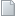Journal of Analysis & Number Theory An International JournalForthcomingThe existence of positive solution for Choquard equations with Hardy-Littlewood-Sobolev critical exponents and Hardy-type term Abstract : We consider the following nonlinear Choquard equation with Dirichlet boundary condition. which involves the critical Sobolev exponent in the sense of the Hardy-Littlewood-Sobolev inequality and Hardy-type terms is considered. Under suitable assumptions on the function f and the parameter \mu>0, we are able to prove the existence of positive solutions. We achieve our goal by making use of variational methods. More specifically, the Mountain Pass Theorem.CERTAIN SUBCLASS OF ANALYTIC UNIVALENT FUNCTIONS USING q􀀀 DIFFERENTIAL OPERATOR Abstract : In this paper, we de fine a new subclass of analytic univalent function using q - differential operator, which generalizes Ruschewayh differential operators. Coefficient inequalities, Subordination, extreme points and integral means inequalities results are obtained.Oscillation of second-order nonlinear neutral dynamic equations with "Maxima" on time scales. Abstract : In this paper, we establish some new oscillation criteria for second order nonlinear neutral delay dynamic equations with "Maxima" on a time scale T. The present results not only generalize and extend some existing results but also can be applied to some of the oscillation problems that are not covered before. Finally, we give some examples to illustrate our main results.On similar transposed sets of polynomials of two complex variables Abstract : This paper studies the effectiveness of similar transposed sets of polynomials of two complex variables in closed complete Reinhardt domains whenever the constituent sets are effective in open complete Reinhardt domains, some notations and definitions introduced in article (1), in article (2) studies the effectiveness of similar transposed sets of polynomials of two complex variables in closed complete Reinhardt domains when the constituent sets are effective in open complete Reinhardt domains and satisfies the normalizing conditions. Also, study the effectiveness of similar transposed sets of polynomials of two complex variables in closed complete Reinhardt domains when the constituent sets are effective in open complete Reinhardt domains and satisfies the algebraic conditions.Solutions for certain classes of Non-linear Diophantine Eqautions Abstract : ABSTRACT: The main aim of this paper is to introduce a method, to solve certain class of non-Linear Diophantine equations and investigate various properties using the well-known Euler’s theorem and the theory of congruence. Some of the interesting special cases of our main results have been discussed. KEY WORDS: Diophantine Equations, Non-linear Diophantine equations, Euler phi-function, Euler’s theorem.Two Dimensional Laplace Transforms for Solving Systems of Fractional Partial Differential Equations Abstract : Abstract In this work, the authors implemented two dimensional Laplace transform to solve certain in homogenous sub ballistic fractional PDE and homogeneous systems of time fractional heat equations which is a generalization to the problem of thermal effects on fluid flow and also the problem of the effect of a uniform overburden on the passage of a thermal wave and the temperatures in the underlying rock. Constructive examples are also provided.